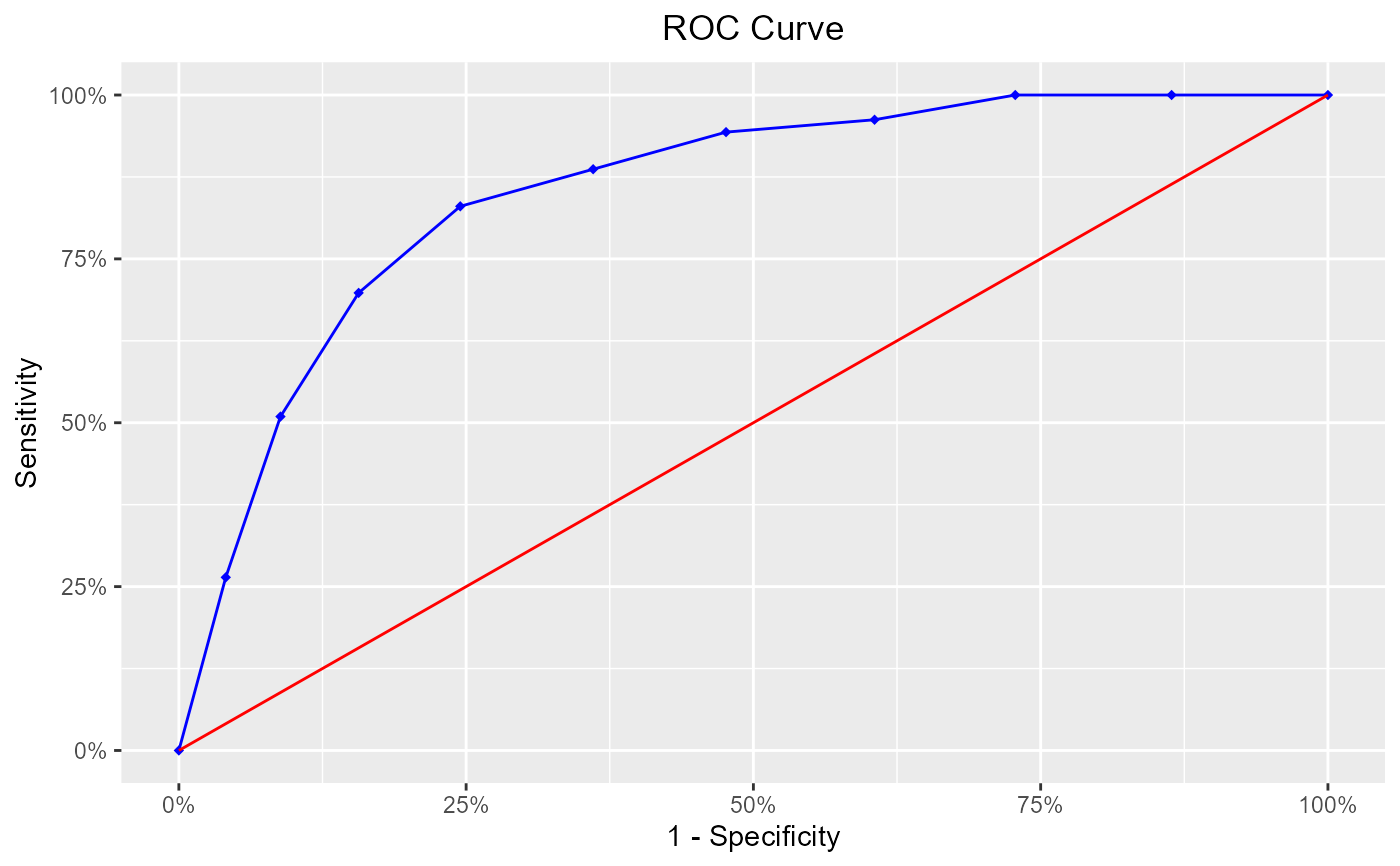Receiver operating characteristic curve (ROC) curve is used for assessing accuracy of the model classification.

blr_roc_curve(
gains_table,
title = "ROC Curve",
xaxis_title = "1 - Specificity",
yaxis_title = "Sensitivity",
roc_curve_col = "blue",
diag_line_col = "red",
point_shape = 18,
point_fill = "blue",
point_color = "blue",
plot_title_justify = 0.5,
print_plot = TRUE
)

## Arguments

gains_table An object of class blr_gains_table. Plot title. X axis title. Y axis title. Color of the roc curve. Diagonal line color. Shape of the points on the roc curve. Fill of the points on the roc curve. Color of the points on the roc curve. Horizontal justification on the plot title. logical; if TRUE, prints the plot else returns a plot object.

Agresti, A. (2007), An Introduction to Categorical Data Analysis, Second Edition, New York: John Wiley & Sons.

Hosmer, D. W., Jr. and Lemeshow, S. (2000), Applied Logistic Regression, 2nd Edition, New York: John Wiley & Sons.

Siddiqi N (2006): Credit Risk Scorecards: developing and implementing intelligent credit scoring. New Jersey, Wiley.

Thomas LC, Edelman DB, Crook JN (2002): Credit Scoring and Its Applications. Philadelphia, SIAM Monographs on Mathematical Modeling and Computation.

Other model validation techniques: blr_confusion_matrix(), blr_decile_capture_rate(), blr_decile_lift_chart(), blr_gains_table(), blr_gini_index(), blr_ks_chart(), blr_lorenz_curve(), blr_test_hosmer_lemeshow()
model <- glm(honcomp ~ female + read + science, data = hsb2,